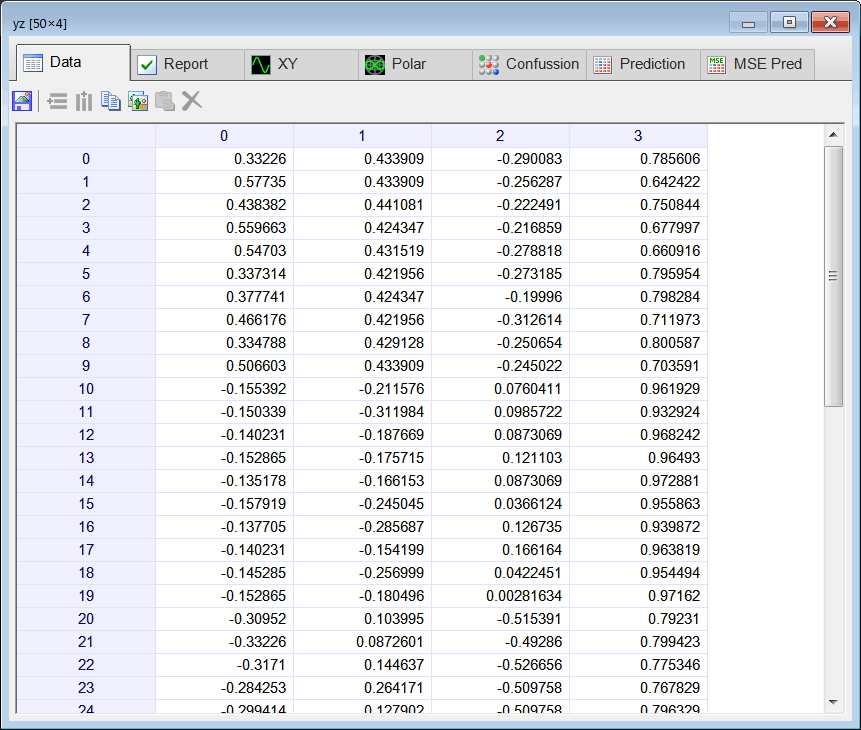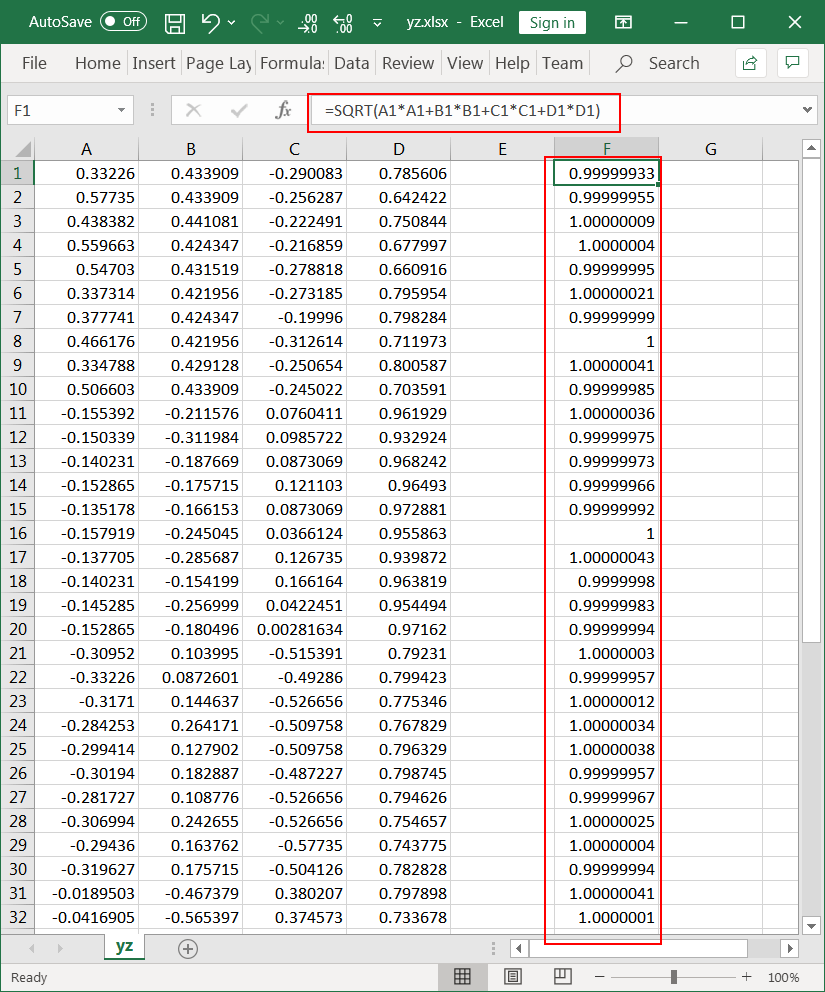# Input Normalization

 Problem 1 An analyst gathered information from 10 people randomly chosen from five countries as shown. Use Microsoft Excel or Notepad to build the file trainSetIn.csv as shown. (Values are for illustrative purposes.)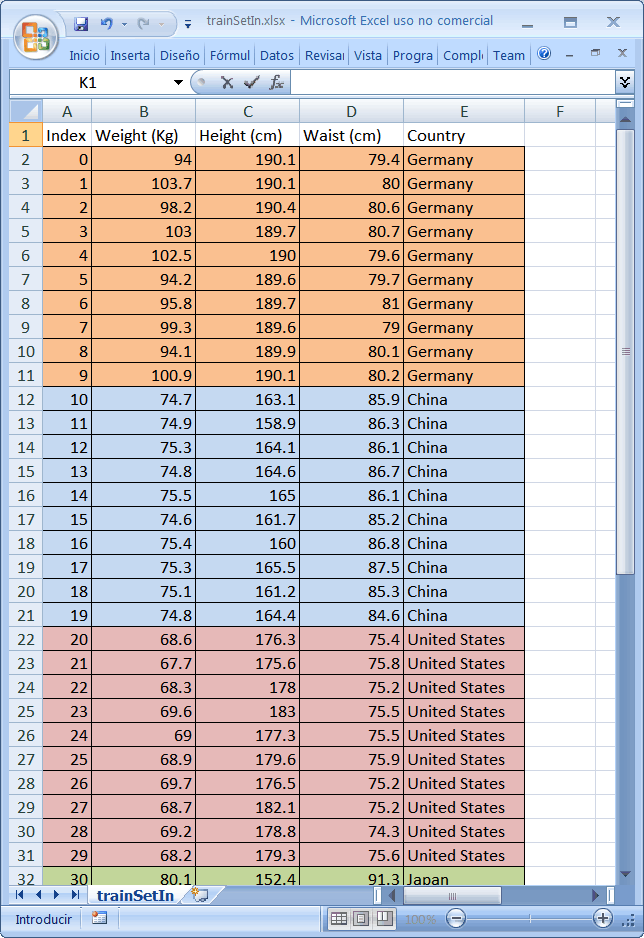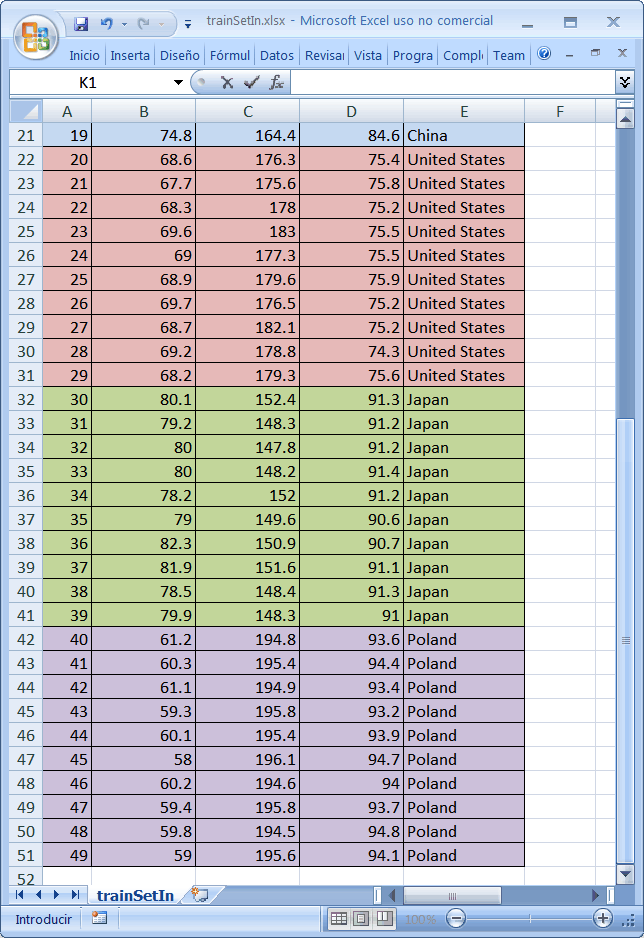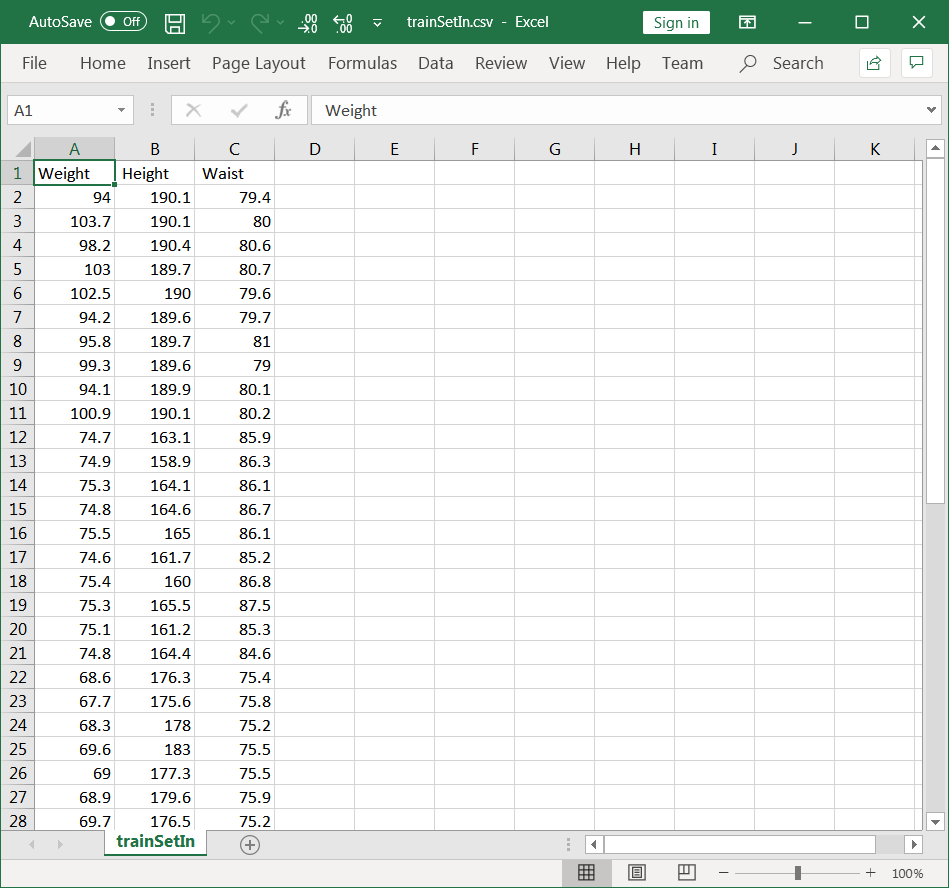Problem 2 Create a Neural Lab project called People; use the Main file only. Load the Main.lab file to load and scale the trainSetIn.csv file. After the scaling the data must be in range from -1 to 1.

 Solution 2A Run the program and double click the trainSetIn to verify that the data was loaded correctly. Use the drop down box to switch the view to Report and Columns as shown. Write down the minimum and maximum values in Column 0, 1, and 2.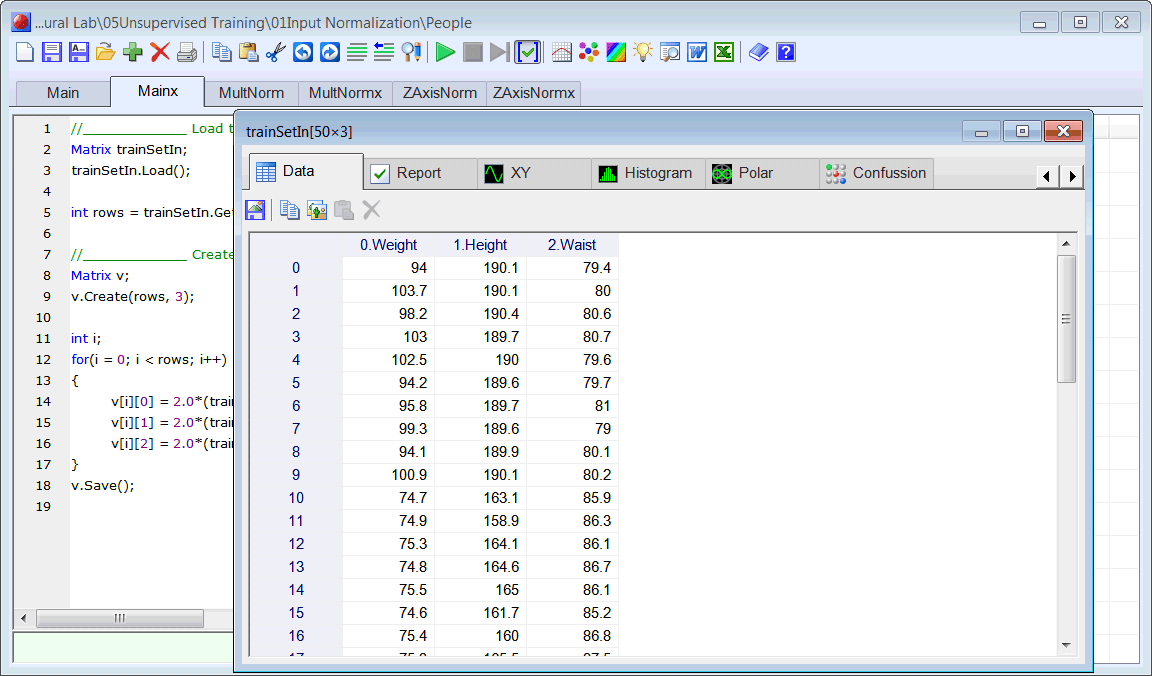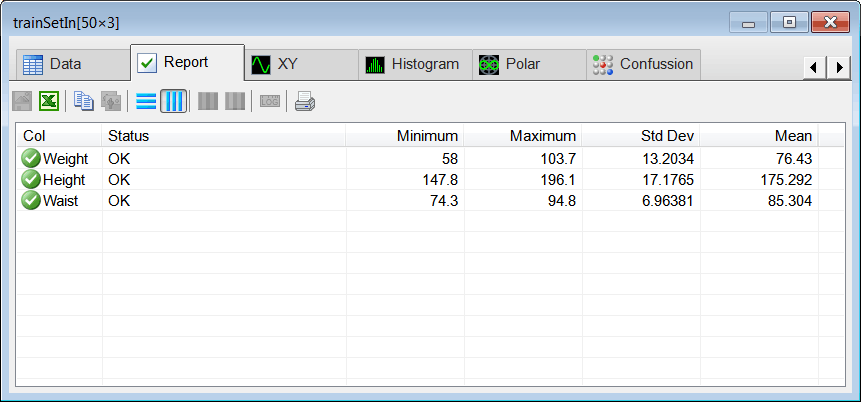Solution 2B Modify and complete the Main.Lab file to produce the scaledInput.csv file. Use the Report to verify that all the values in the variable v are in the range from -1 to 1.

 People\Main.lab //_____________ Load the training set input Matrix trainSetIn; trainSetIn.Load(); int rows = trainSetIn.GetRowCount(); //_____________ Create the Scaled Input Matrix v; v.Create(rows, 3); int i; for(i = 0; i < rows; i++) {      v[i] = ...;      v[i] = ...;      v[i] = ...; } v.Save();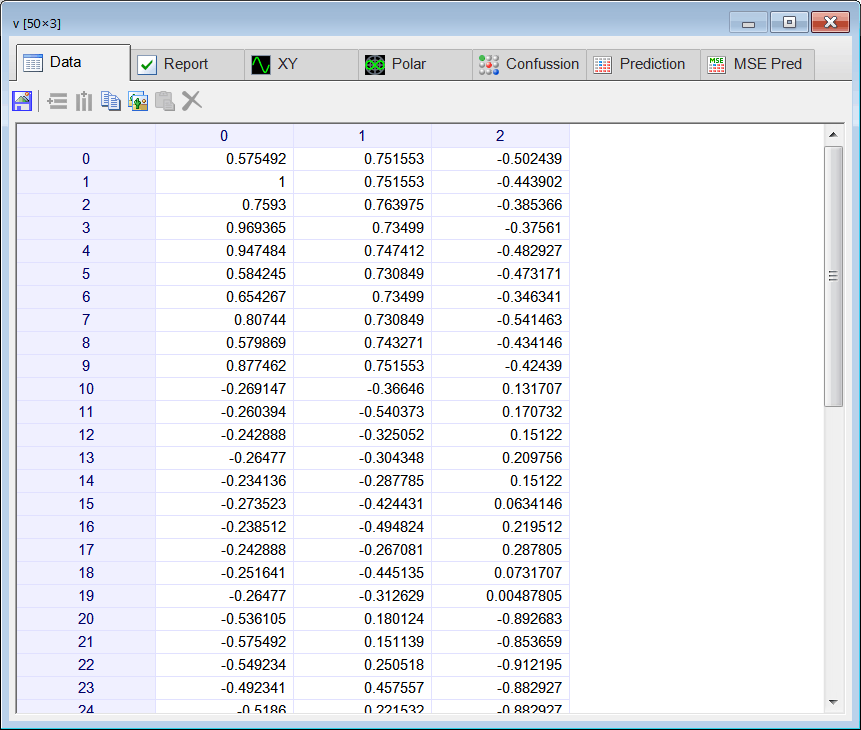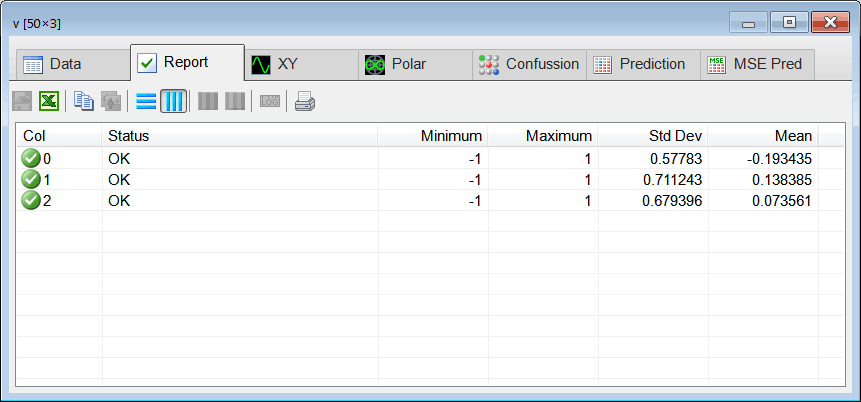## Input Normalization

Input normalization is very important for Kohonen networks. All input values must be in the range [-1 1]. For best performance, the length (usually the vector norm) of each case in the input must be equal to one. There are two types of input normalization: Multiplicative and Z-Axis.

## Multiplicative Normalization

For each input case, the length (vector norm) of the input is computed. Then, that case is divided by its length as shown in the figure below. This method normalizes the input so that each input case has a length (vector norm) of one. This method is commonly used; however one problem that may arise is that some input cases that were originally different may be equal after normalization.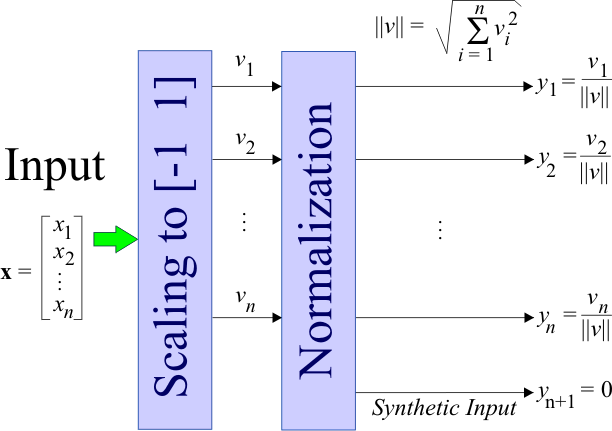Problem 3 Create the file MultNorm.lab to create y the normalized input using multiplicative normalization. Run the program and open the y.csv file using Microsoft Excel (You may select the file from the Neural Lab file list and click the Microsoft Excel button from the toolbar.) Once the file is open in Microsoft Excel insert the formula to compute the vector norm; it must be one.

 People\MultNorm.lab //____________________________ Load the Scaled Input Matrix v; v.Load(); //___________________________ Normalize int rows = v.GetRowCount(); int cols = v.GetColCount(); Matrix y; y.Create(rows, cols); int i; int j; double norm; for(i = 0; i < rows; i++) {      //______________________ Compute the norm      ...      //______________________ Compute y      ... } y.Save();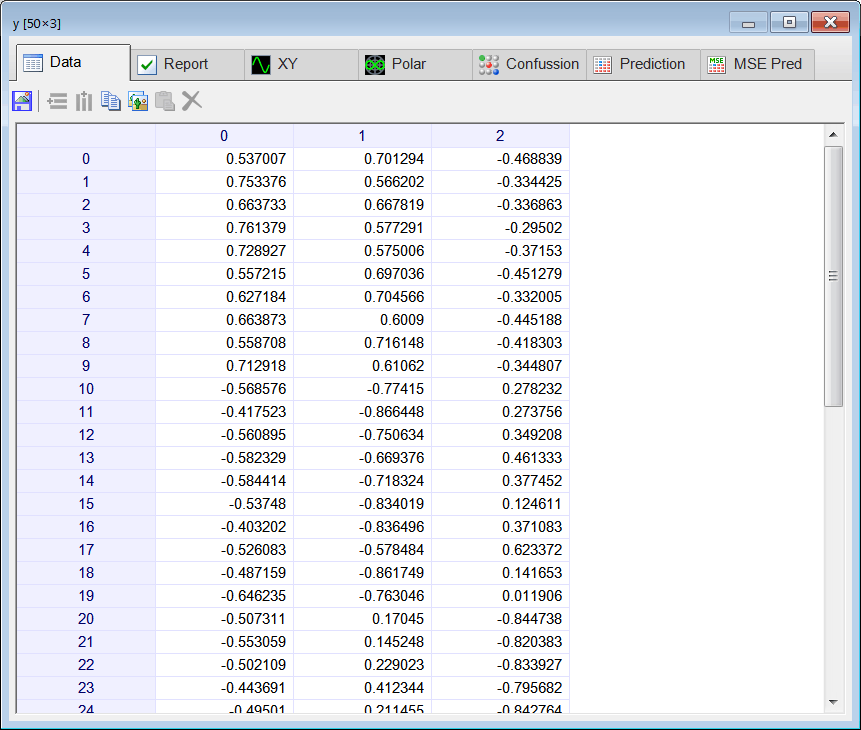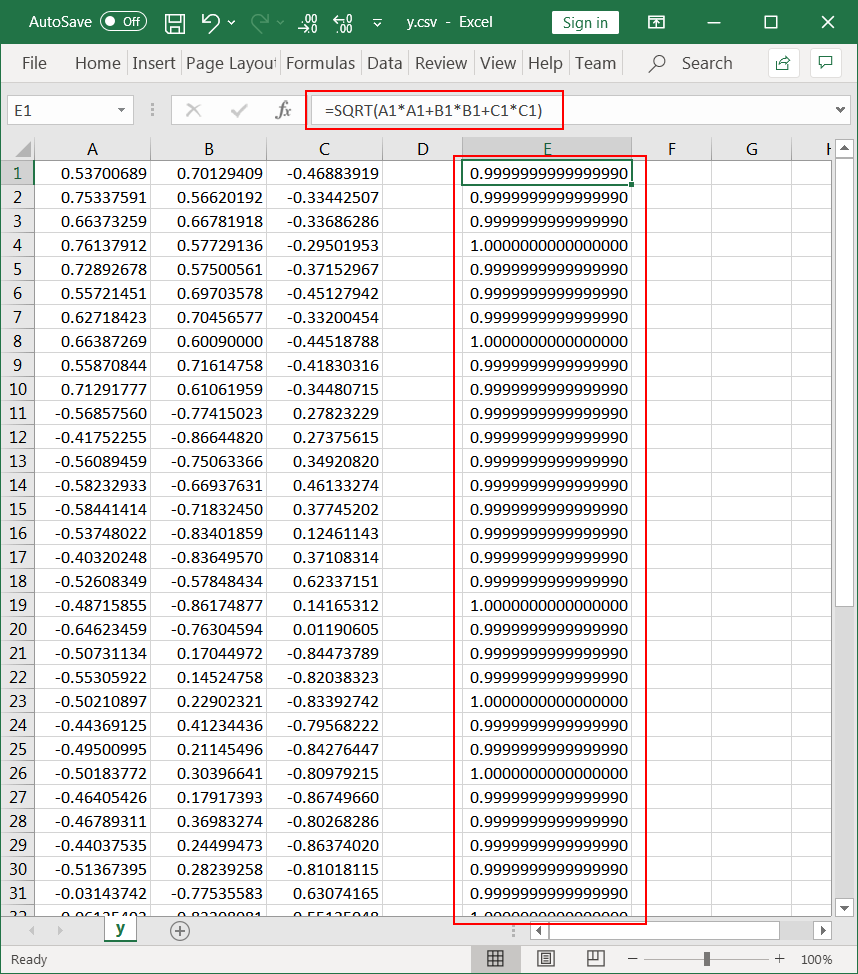## Z-Axis Normalization

Z-Axis normalization consists by adding a synthetic value to the input to change the length (vector norm) of the input. First, each input value is divided by the squared root of the number of inputs. Second, the synthetic value is computed and added to the original input. The figure below shows how Z-Axis normalization is performed. The Z-Axis normalization method is very popular; however, if most input values are almost zero, the synthetic input value will be high creating a numerical stability problem. In these cases, Multiplicative normalization must be used.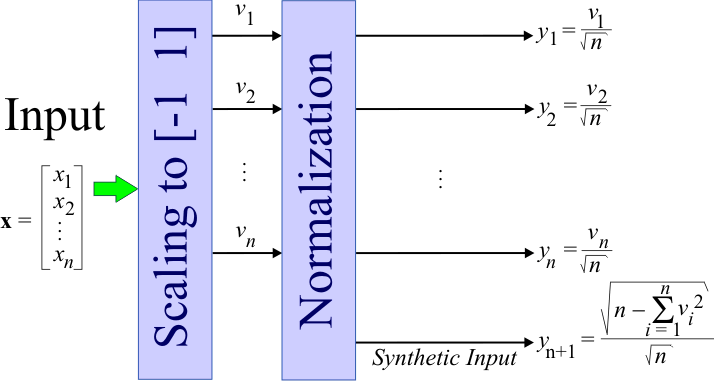Problem 4 Create the file ZAxisNorm.lab to create y the normalized input using Z-Axis normalization. Run the program and open the yz.csv file using Microsoft Excel (You may select the file from the Neural Lab file list and click the Microsoft Excel button from the toolbar.) Once the file is open in Microsoft Excel insert the formula to compute the vector norm; it must be one.

 People\ZAxisNorm.lab //____________________________ Load the Scaled Input Matrix v; v.Load(); //___________________________ Normalize int rows = v.GetRowCount(); int cols = v.GetColCount(); Matrix yz; yz.Create(rows, cols+1); int i; int j; double sumSquares; for(i = 0; i < rows; i++) {      //______________________ Compute y      ...      //______________________ Compute sum of v*v      ...      //_____________________ Compute Synthetic input      ... } yz.Save();